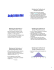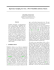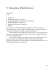# Sampling Distributions

## Transcription

Sampling Distributions
```Sampling Distributions
Formulas
New Vocabulary
•
Proportions summarize categorical variables.
•
The Normal sampling distribution model looks like it will be very useful.
•
Can we do something similar with quantitative data?
•
We can indeed. Even more remarkable, not only can we use all of the same
concepts, but almost the same model.
•
Like any statistic computed from a random sample, a sample mean also has a
sampling distribution.
•
We can use simulation to get a sense as to what the sampling distribution of the
sample mean might look like…
Means – The “Average” of One Die
•
10,000 tosses of a die. A
histogram of the results is:
Means – Averaging More Dice
•
Looking at the average of two dice after
a simulation of 10,000 tosses:
•
The average of three dice after a
simulation of 10,000 tosses looks like:
•
The average of 5 dice after a simulation
of 10,000 tosses looks like:
•
The average of 20 dice after a
simulation of 10,000 tosses looks like:
Means – What the Simulations Show
•
As the sample size (number of dice) gets larger, each sample average is more
likely to be closer to the population mean.
•
And, the sampling distribution of a mean becomes more Normal.
The Fundamental Theorem of Statistics
•
The sampling distribution of any mean becomes more Normal as the sample
size grows.
–
All we need is for the observations to be independent and collected with
randomization.
–
•
We don’t even care about the shape of the population distribution!
The Fundamental Theorem of Statistics is called the Central Limit Theorem
(CLT).
•
The CLT is surprising and a bit weird:
–
Not only does the histogram of the sample means get closer and closer
to the Normal model as the sample size grows, but this is true
regardless of the shape of the population distribution.
•
The CLT works better (and faster) the closer the population model is to a
Normal itself. It also works better for larger samples.
The Central Limit Theorem (CLT)
The mean of a random sample has a sampling distribution whose shape can be
approximated by a Normal model. The larger the sample, the better the approximation
will be.
•
The CLT requires remarkably few assumptions, so there are few conditions to
check:
–
Random Sampling Condition: The data values must be sampled
randomly or the concept of a sampling distribution makes no sense.
–
Independence Assumption: The sample values must be mutually
independent. (When the sample is drawn without replacement, check
the 10% condition…)
•
The population mean for the sampling distribution is equal to the population
mean.
•
The standard deviation of the sampling distribution for means is equal to the
population standard deviation divided by the square root of the sample size.
•
The standard deviation of the sampling distribution declines only with the
square root of the sample size.
•
While we’d always like a larger sample, the square root limits how much we
can make a sample tell about the population. (This is an example of the Law of
Diminishing Returns.)
Example:
The mean annual income for adult
women in one city is \$28,520 and
the standard deviation of the
incomes is \$5700. The
distribution of incomes is skewed
to the right. Determine the
sampling distribution of the mean
for samples of size 110. In
particular, state whether the
distribution of the sample mean is
normal or approximately normal
and give its mean and standard
deviation.
Example:
The number of hours per week
that high school seniors spend on
homework is normally distributed,
with a mean of 11 hours and a
standard deviation of 3 hours. 70
students are chosen at random.
Find the probability that the mean
number of hours spent on
homework for this group is
between 10.2 and 11.5.
Example:
At a shoe factory, the time taken
to polish a finished shoe has a
mean of 3.7 minutes and a
standard deviation of 0.48
minutes. If 44 shoes are polished,
there is a 5% chance that the mean
time to polish the shoes is below
what value?
•
Both of the sampling distributions we’ve looked at are Normal.
–
For proportions
– For means
•
We will use sample statistics to estimate these population parameters.
•
Whenever we estimate the standard deviation of a sampling distribution, we call
it a standard error.
• For a sample proportion, the
standard error is
• For the sample mean, the
standard error is
Sampling Distribution Models
•
Always remember that the statistic itself is a random quantity.
–
We can’t know what our statistic will be because it comes from a
random sample.
•
Fortunately, for the mean and proportion, the CLT tells us that we can model
their sampling distribution directly with a Normal model.
•
There are two basic truths about sampling distributions:
–
Sampling distributions arise because samples vary. Each random
sample will have different cases and, so, a different value of the
statistic.
–
Although we can always simulate a sampling distribution, the Central
Limit Theorem saves us the trouble for means and proportions.
SUMMARY
```

### Email: [email protected]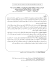### SAMPLING DISTRIBUTIONS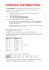### Chapter 11. Sampling Distributions. Problems and definitions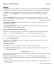### ( 3) log ( ) xxy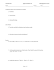### 6734-2 CS6000 Leaflet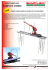### Chapter 12: Sample Surveys Terms and Notes Sample: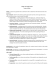### 7a. Fish Diversity Survey in Teluk Rubiah (English - pdf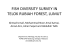### 4.1: Sampling &amp; Surveys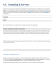### Chapter 7 Sample Variability Statistics I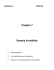### South Towne Expo Center - The Great Salt Lake Business Conference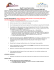### South Towne Expo Center and Salt Palace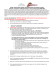### Document 6534743